#### Outliers

In data analysis the identification of outliers, meaning observations that fall well outside the overall pattern of the data, is very important. An outlier requires special attention. It may be the result of a measurement or recording error, an observation from a different population or an unusual extreme observation. Note that an extreme observation does not need to be an outlier; it can instead be an indication of skewness (Weiss 2010)).

If we observe an outlier, we should try to determine its cause. If an outlier is caused by a measurement or recording error or if for some other reason it clearly does not belong to the data set, the outlier can simply be removed. However, if no explanation for an outlier is apparent, the decision whether to retain it in the data set is a difficult judgment call.

As a diagnostic tool for spotting observations that may be outliers we may use quartiles and the $$IQR$$. For this we define the lower limit and the upper limit of a data set. The lower limit is the number that lies $$1.5 \times IQRs$$ below the first quartile; the upper limit is the number that lies $$1.5 \times IQRs$$ above the third quartile. Observations that lie below the lower limit or above the upper limit are potential outliers (Weiss 2010).

$\text{Lower limit} = Q1 - 1.5 \times IQR$ $\text{Upper limit} = Q3 + 1.5 \times IQR$

#### Boxplots

A boxplot, also called a box-and-whisker diagram, is based on the five-number summary and can be used to provide a graphical display of the center and variation of a data set. These diagrams were invented by the mathematician John Wilder Tukey. Several types of boxplots are in common use.

Box-and-whisker plots give a graphic representation of data using five measures: the median, the first quartile, the third quartile as well as the smallest and the largest value between the lower and the upper limits of the data set. The spacing between the different parts of the box indicates the degree of dispersion (spread) and skewness in the data. We can compare different distributions by making box-and-whisker plots for each of them. It also helps to detect outliers (Mann 2012). Box plots can be drawn either horizontally or vertically.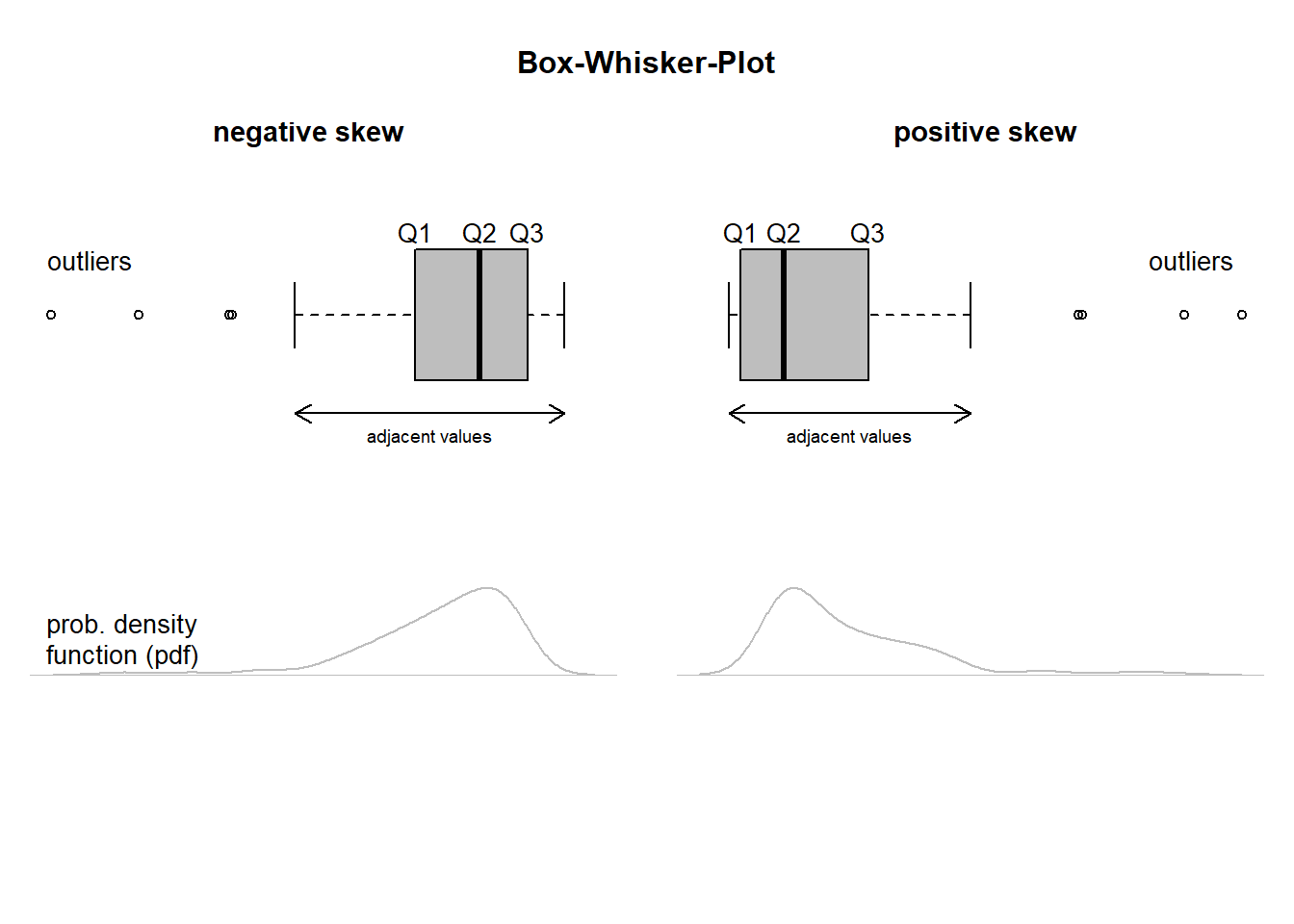The edges of the box are always the first and third quartile, and the band inside the box is always the second quartile (the median). The lines extending from the boxes (whiskers) indicate the variability outside the upper and lower quartile. To construct a boxplot, we also need the concept of adjacent values. The adjacent values of a data set are the most extreme observations that still lie within the lower and upper limits; i.e. they are the most extreme observations that are not potential outliers. Outliers may be plotted as individual points. Note that, if a data set has no potential outliers, the adjacent values are just the minimum and maximum observations (Weiss 2010).

Let us now construct a series of boxplots in order to analyze the students data set in more depth. We start by constructing a boxplot for the nc.score variable.

students <- read.csv("https://userpage.fu-berlin.de/soga/data/raw-data/students.csv")
boxplot(students$nc.score)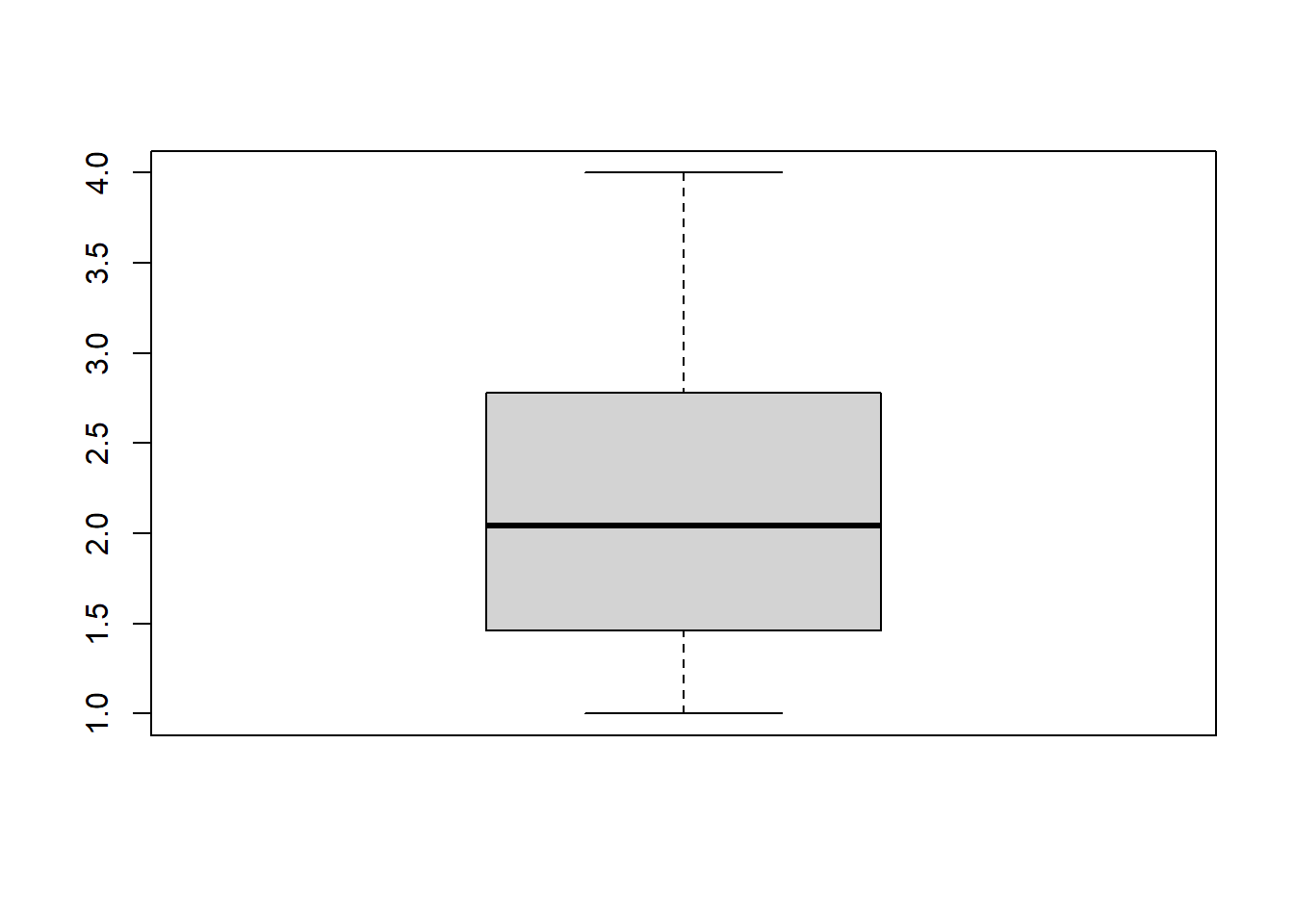We immediately get an impression of the spread and skewness in the data. By adding the argument horizontal = TRUE to the boxplot we rotate the boxplot by 90$$^{\circ}$$. For the sake of a better visual impression we also colorize the box. boxplot(students$nc.score,
col = "blue",
horizontal = TRUE,
xlab = "Scores",
main = "Numerus clausus"
)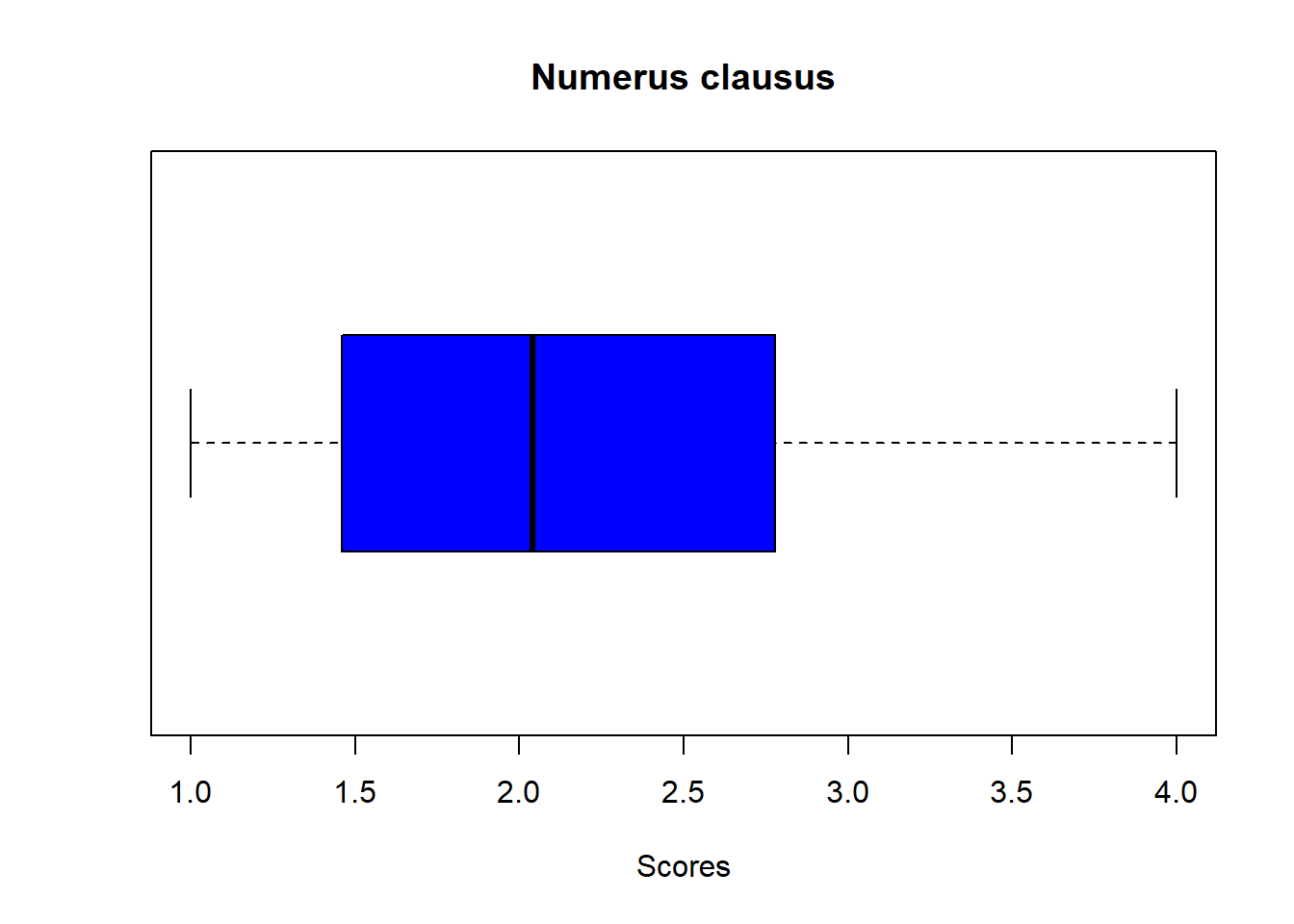Boxplots are a very powerful technique for exploratory data analysis as it is very easy to condition the variable of interest, in our case the nc.score variable, on other variables. In R we condition one variable on another by using the ~ (this is the so called formula notation).

Let us plot a boxplot of the nc.score variable conditioned on the semester variable. The semester variable corresponds to the semester the particular student is studying. For your information: The minimum period of study for the study programs under investigation is set to 4 semesters.

par(mar = c(2.5, 5, 5.8, 3))
boxplot(students$nc.score ~ students$semester,
col = "blue",
horizontal = TRUE,
xlab = "Scores",
ylab = "Semester",
main = "Numerus clausus for students of\n different semesters"
)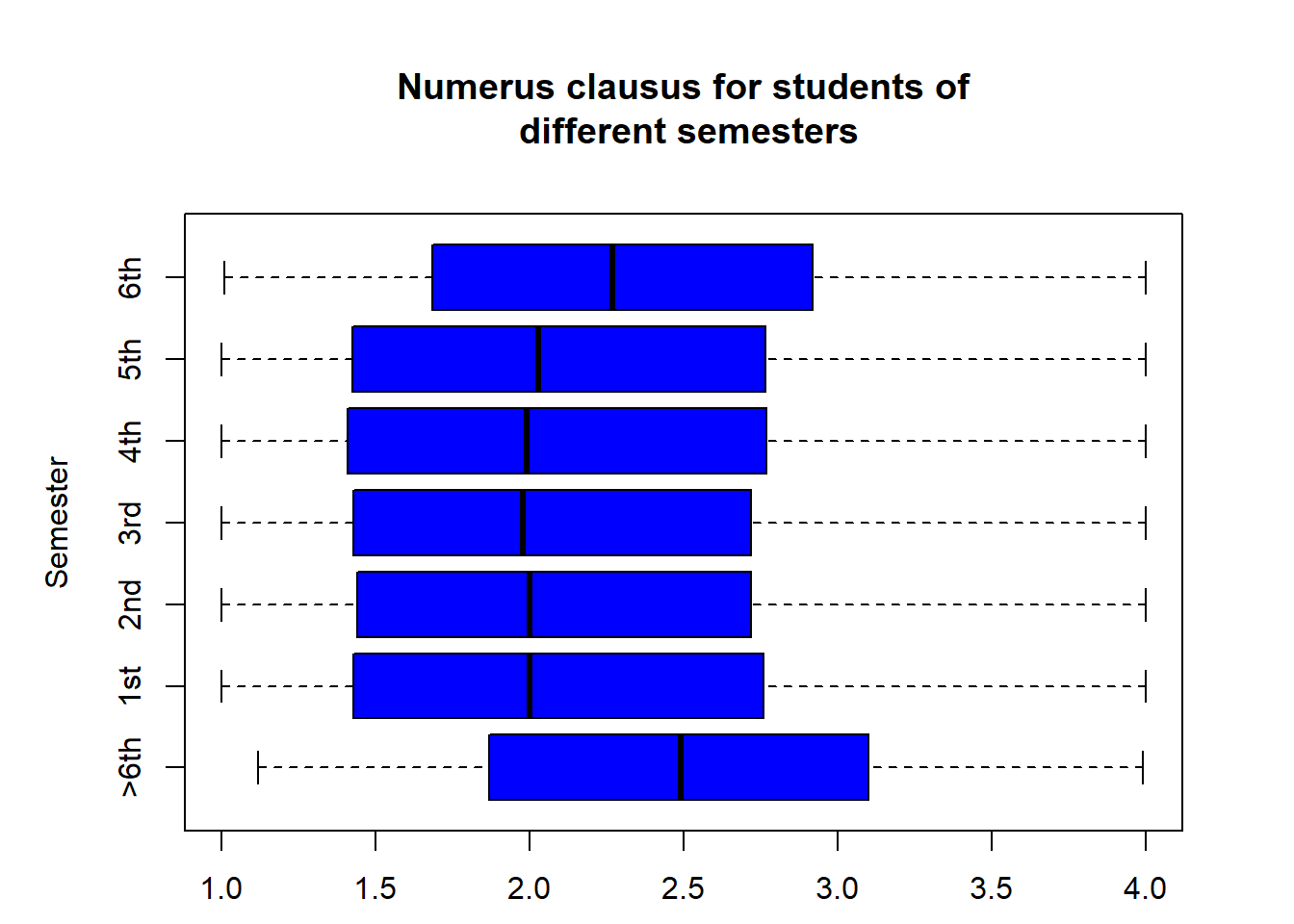Interesting, isn’t it? The plot suggests that students of higher semesters (> 5th) tend do score lower on the numerus clausus. Or, in other words, those students who finish their studies within the minimum period of study tend to have a higher numerus clausus score.

Still, we are not yet finished. We want to know whether gender has any effect on that observation. We can easily incorporate an interaction variable by simply adding the variable with a + sign. In addition, we introduce the notch argument. If the notches of two plots do not overlap this is “strong evidence” that the two medians differ (Chambers, et al. (1983): Graphical Methods for Data Analysis. Wadsworth & Brooks/Cole, p. 62). For further information type help(boxplot) or help(boxplot.stats) in your console. Please be aware, that in order to get a nicer looking y-axis we must write one additional line of code.

par(mar = c(2.5, 5, 5.8, 3), xpd = TRUE)
boxplot(students$nc.score ~ students$gender + students$semester, col = c("blue", "red"), horizontal = TRUE, notch = T, xlab = "Numerus clausus scores", ylab = "Semester", yaxt = "n", main = "Numerus clausus for students of\n different semesters and gender" ) # add a legend legend( x = 2, y = 16.6, legend = c("Female", "Male"), col = c("blue", "red"), pch = 15, bty = "n", pt.cex = 3, cex = 1, horiz = T ) # add the y axis label axis(2, at = seq(1.5, 14, 2), labels = unique(students$semester), tick = T)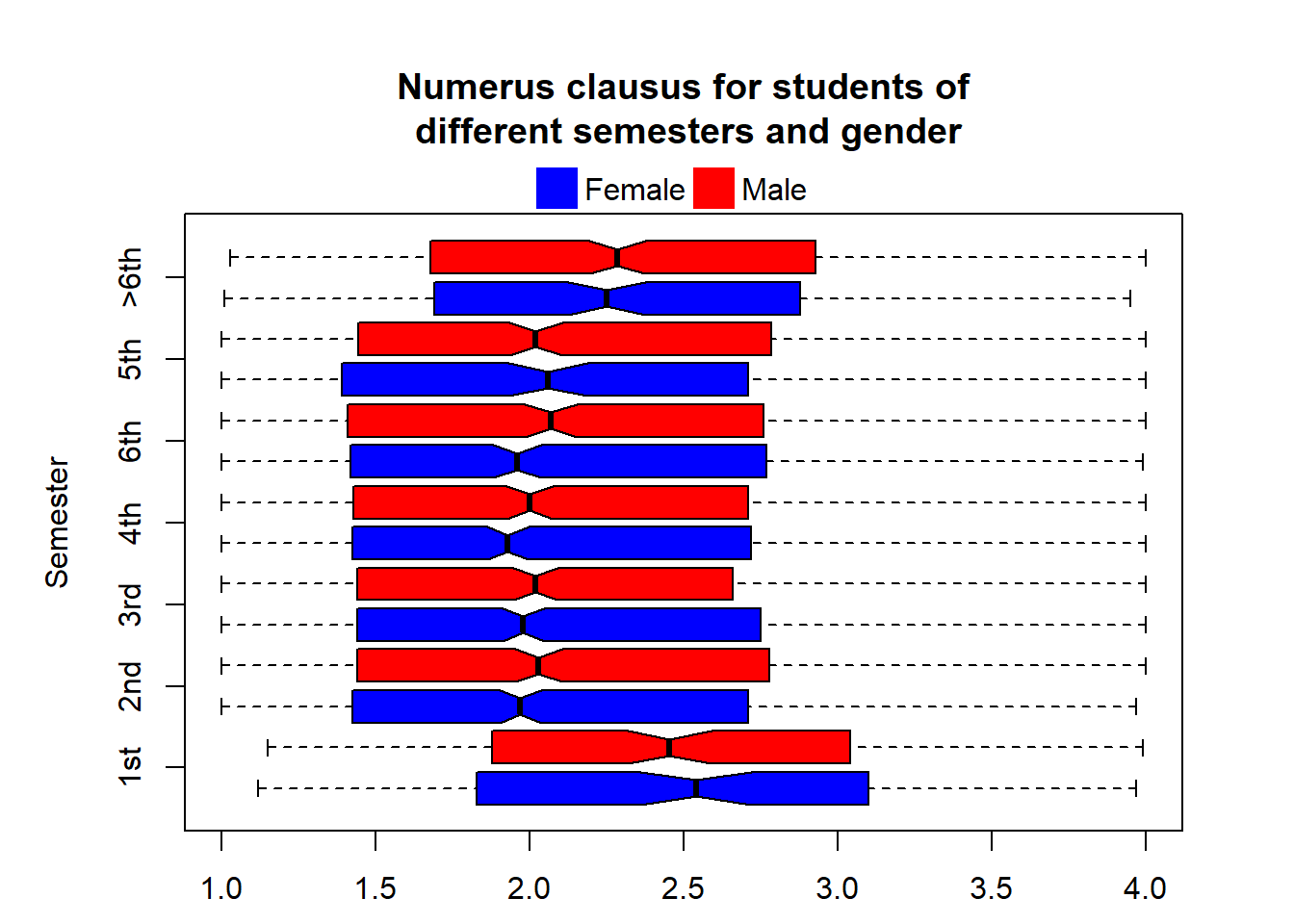This plot is not as easy to interpret. Though, it seems that the observation we made previously is confirmed: students of higher semesters (> 5th) tend do score a lower numerus clausus. However, the impact of gender on the numerus clausus scores is not as clear. We will have to apply methods of inferential statistics to assess whether these differences are statistically significant or whether these fluctuations around the median may also be caused solely by chance.

To wrap this section up and in order to see a boxplot with outliers too, we plot the height variable against the gender variable. This time we use the extremely powerful ggplot2 package for advanced plotting. You can use all ggplot2 functions by calling install.packages("ggplot2") and attaching it to the workspace by calling library(ggplot2).

library(ggplot2)
ggplot(students, aes(gender, height, fill = gender)) +
geom_boxplot(outlier.color = "red", outlier.shape = 6, outlier.size = 1.5, width = 0.9) +
geom_line(color = "#3366FF", alpha = 0.5) +
labs(title = "The height of students based on gender", x = "", y = "Height in cm") +
scale_fill_manual(name = "Legend", values = c("#fc8d59", "#91bfdb")) +
theme_minimal()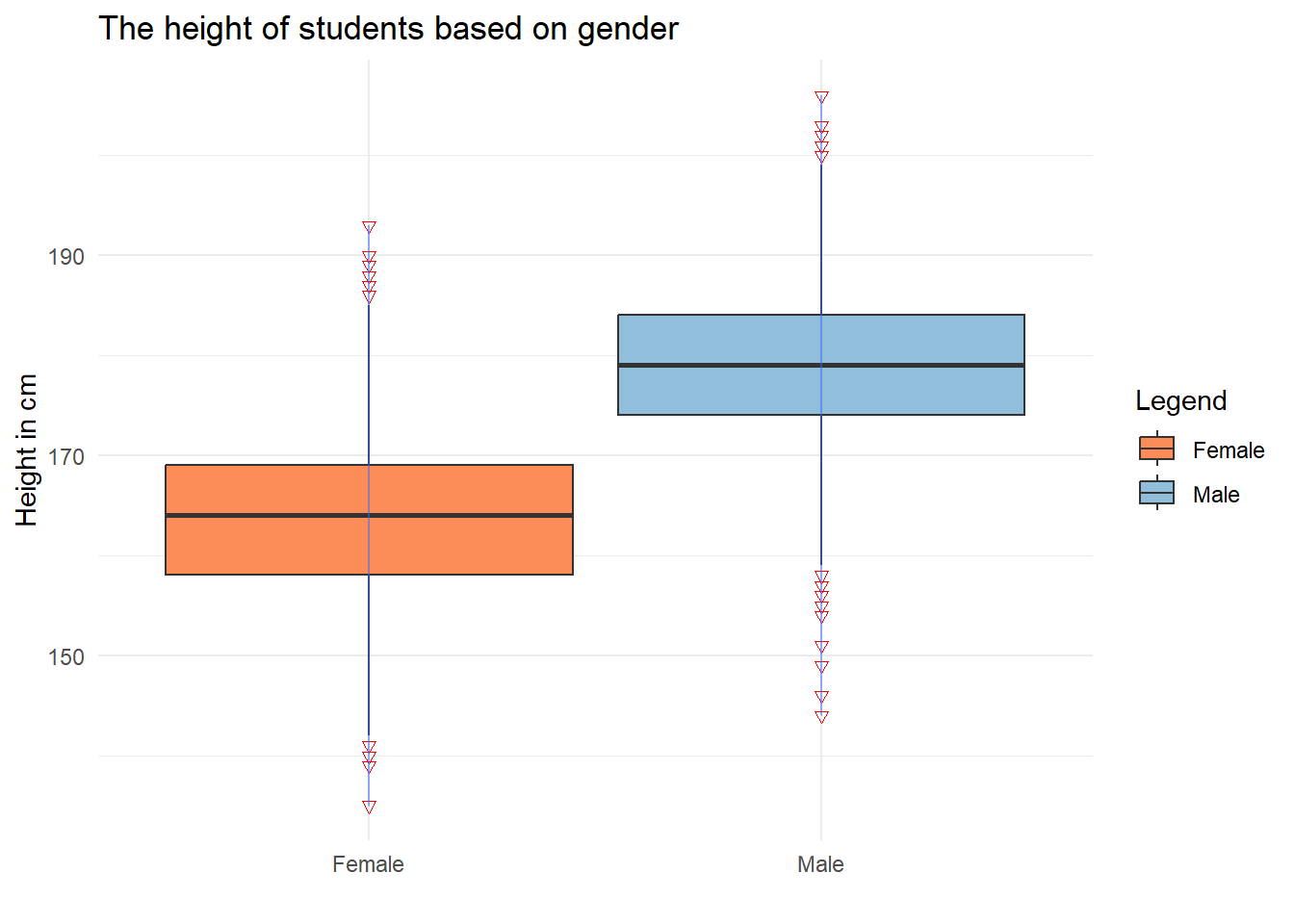Obviously, and certainly not that unexpectedly, there is a difference in the height of the students among the different groups (male or female). Female students tend to be smaller than male students, but, if we look at the extremes, there are tall and short individuals in both groups. However, as mentioned above, we will have to test our observations for statistical significance to be more confident, that the observed difference in height is not just there by chance.

Citation

The E-Learning project SOGA-R was developed at the Department of Earth Sciences by Kai Hartmann, Joachim Krois and Annette Rudolph. You can reach us via mail by soga[at]zedat.fu-berlin.de.You may use this project freely under the Creative Commons Attribution-ShareAlike 4.0 International License.

Please cite as follow: Hartmann, K., Krois, J., Rudolph, A. (2023): Statistics and Geodata Analysis using R (SOGA-R). Department of Earth Sciences, Freie Universitaet Berlin.